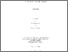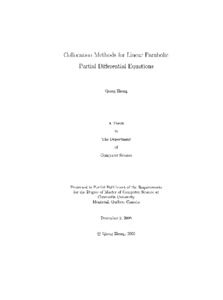Title:

# Collocation methods for linear parabolic partial differential equations

Zheng, Qiang (2005) Collocation methods for linear parabolic partial differential equations. Masters thesis, Concordia University.Preview
Text (application/pdf)
MR14342.pdf - Accepted Version
8MB

## Abstract

This thesis presents a new class of collocation methods for the approximate numerical solution of linear parabolic partial differential equations. In the time dimension, the partial derivative with respect to time is replaced by finite differences, to form the implicit Euler method. At each time step, a polynomial approximating the exact solution is calculated for each triangular finite element created by the Rivara algorithm. Polynomials of adjacent finite elements have matching values and matching normal derivatives at a set of discrete points, called "matching points". The method of nested dissection is used to eliminate all variables at the interior matching points of the domain. The maximum error of the solution is of the order of the time step size, which is O ( dt ), except when dt is sufficiently small. In that case, the maximum error can be very small, depending on the density of the space mesh. An application based on OpenGL and Motif to visualize the solutions is also described in this thesis. Extensive numerical results, pictures of refined meshes, and 3 D representations of the solutions are given

Divisions: Concordia University > Gina Cody School of Engineering and Computer Science > Computer Science and Software Engineering Thesis (Masters) Zheng, Qiang xiii, 126 leaves : ill. ; 29 cm. Concordia University M. Comp. Sc. Computer Science and Software Engineering 2005 Doedel, Eusebius 8793 Concordia University Library 18 Aug 2011 18:35 18 Jan 2018 17:34 http://clues.concordia.ca/search/c?SEARC...
All items in Spectrum are protected by copyright, with all rights reserved. The use of items is governed by Spectrum's terms of access.

Repository Staff Only: item control page

Back to top